# 11 2 Surface Area of Prisms and Cylinders

• Slides: 32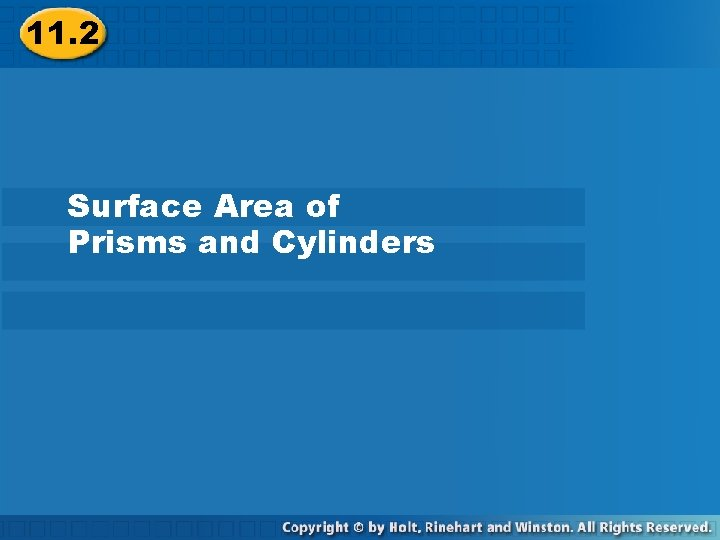11. 2 Surface Area of Prisms and Cylinders11. 2 Surface Area of Prisms and Cylinders Prisms and cylinders have 2 congruent parallel bases. A lateral face is not a base. The edges of the base are called base edges. A lateral edge is not an edge of a base. The lateral faces of a right prism are all rectangles. An oblique prism has at least one nonrectangular lateral face.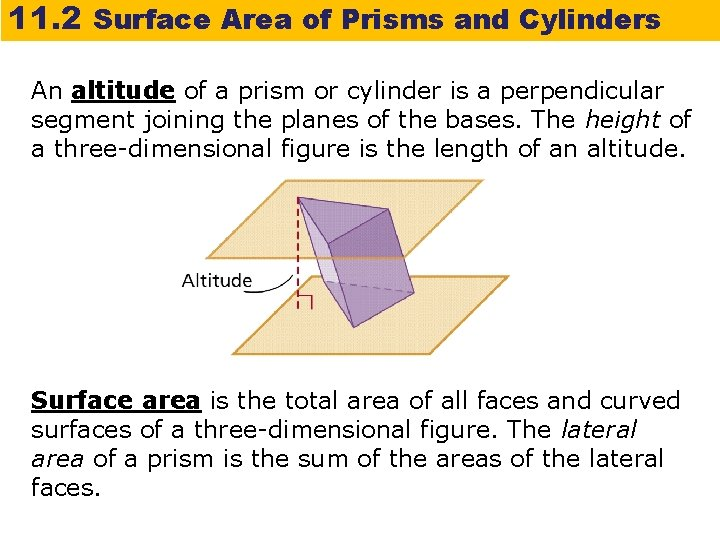11. 2 Surface Area of Prisms and Cylinders An altitude of a prism or cylinder is a perpendicular segment joining the planes of the bases. The height of a three-dimensional figure is the length of an altitude. Surface area is the total area of all faces and curved surfaces of a three-dimensional figure. The lateral area of a prism is the sum of the areas of the lateral faces.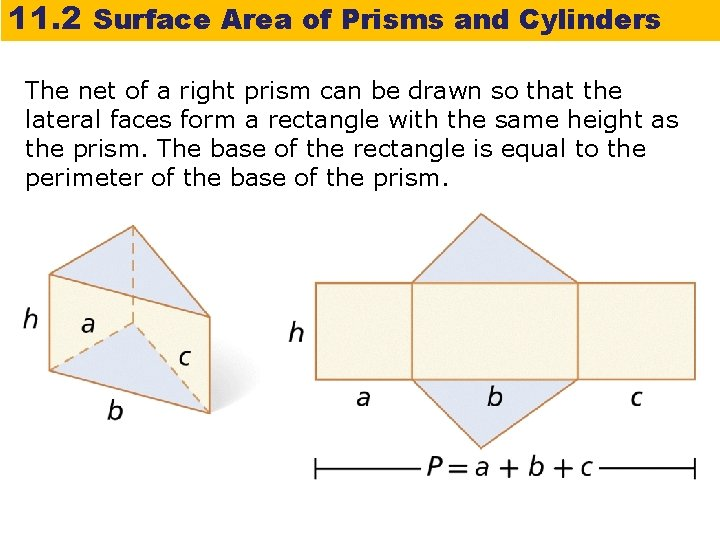11. 2 Surface Area of Prisms and Cylinders The net of a right prism can be drawn so that the lateral faces form a rectangle with the same height as the prism. The base of the rectangle is equal to the perimeter of the base of the prism.11. 2 Surface Area of Prisms and Cylinders The surface area of a right rectangular prism with length ℓ, width w, and height h can be written as S = 2ℓw + 2 wh + 2ℓh.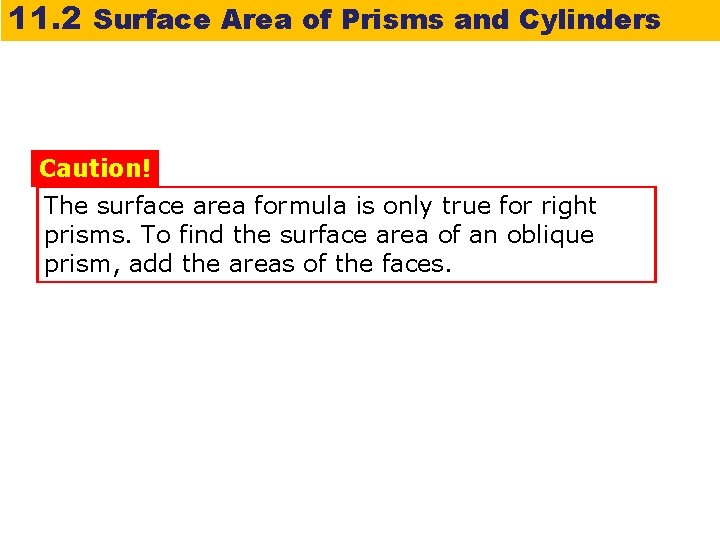11. 2 Surface Area of Prisms and Cylinders Caution! The surface area formula is only true for right prisms. To find the surface area of an oblique prism, add the areas of the faces.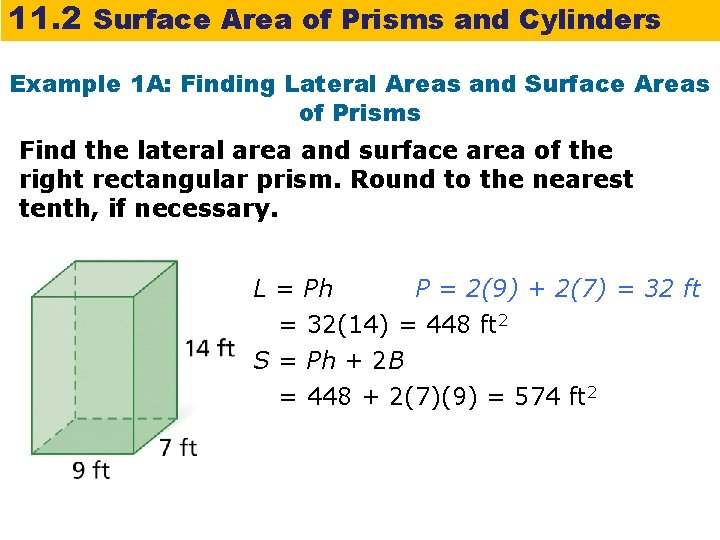11. 2 Surface Area of Prisms and Cylinders Example 1 A: Finding Lateral Areas and Surface Areas of Prisms Find the lateral area and surface area of the right rectangular prism. Round to the nearest tenth, if necessary. L = Ph P = 2(9) + 2(7) = 32 ft = 32(14) = 448 ft 2 S = Ph + 2 B = 448 + 2(7)(9) = 574 ft 2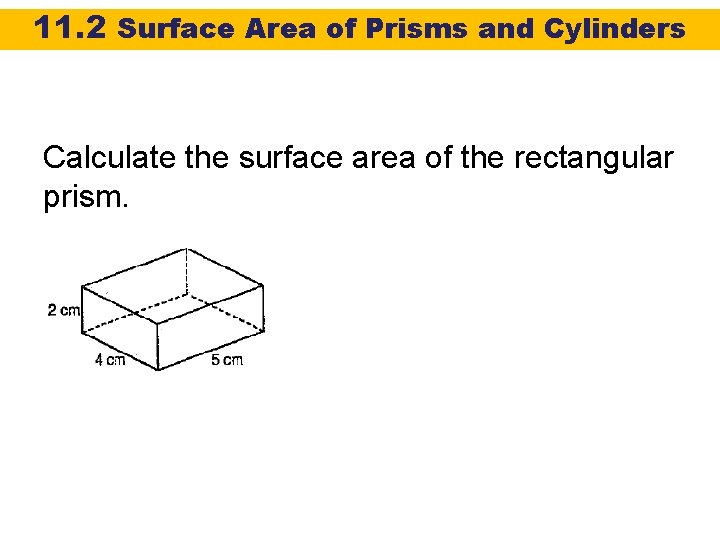11. 2 Surface Area of Prisms and Cylinders Calculate the surface area of the rectangular prism.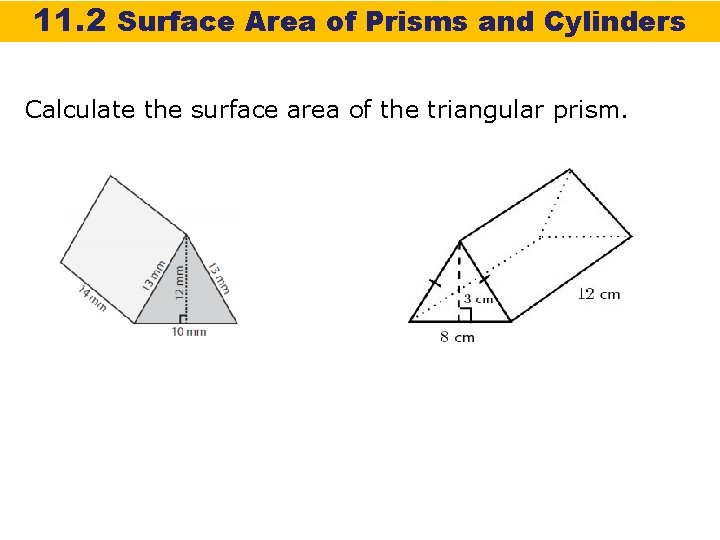11. 2 Surface Area of Prisms and Cylinders Calculate the surface area of the triangular prism.11. 2 Surface Area of Prisms and Cylinders Check It Out! Example 1 Find the lateral area and surface area of a cube with edge length 8 cm. L = Ph = 32(8) = 256 cm 2 P = 4(8) = 32 cm S = Ph + 2 B = 256 + 2(8)(8) = 384 cm 2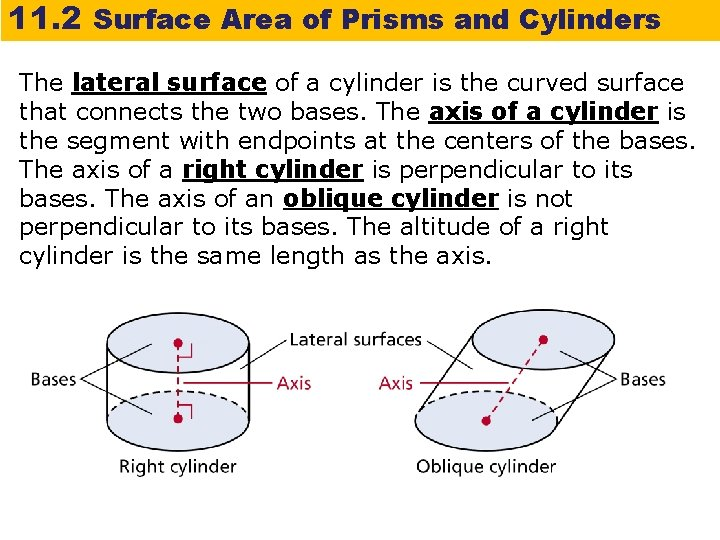11. 2 Surface Area of Prisms and Cylinders The lateral surface of a cylinder is the curved surface that connects the two bases. The axis of a cylinder is the segment with endpoints at the centers of the bases. The axis of a right cylinder is perpendicular to its bases. The axis of an oblique cylinder is not perpendicular to its bases. The altitude of a right cylinder is the same length as the axis.11. 2 Surface Area of Prisms and Cylinders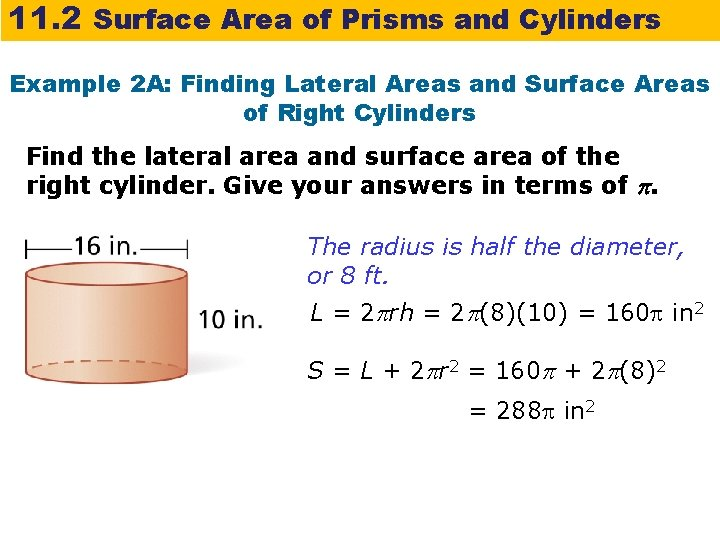11. 2 Surface Area of Prisms and Cylinders Example 2 A: Finding Lateral Areas and Surface Areas of Right Cylinders Find the lateral area and surface area of the right cylinder. Give your answers in terms of . The radius is half the diameter, or 8 ft. L = 2 rh = 2 (8)(10) = 160 in 2 S = L + 2 r 2 = 160 + 2 (8)2 = 288 in 2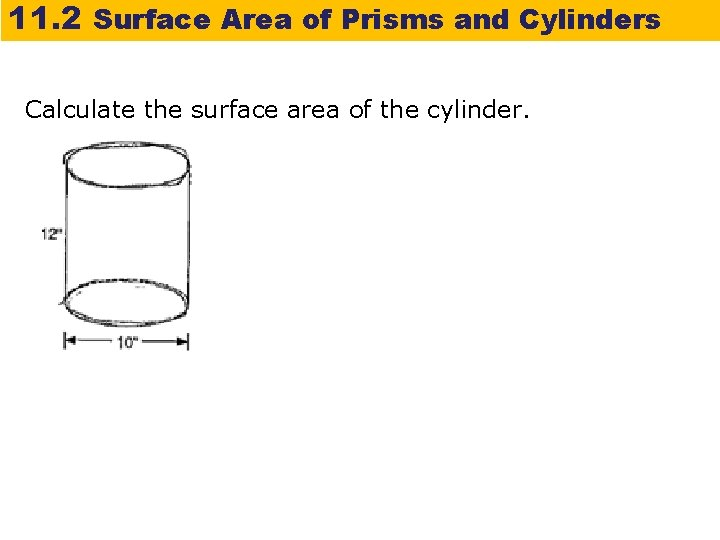11. 2 Surface Area of Prisms and Cylinders Calculate the surface area of the cylinder.11. 2 Surface Area of Prisms and Cylinders Example 2 B: Finding Lateral Areas and Surface Areas of Right Cylinders Find the lateral area and surface area of a right cylinder with circumference 24 cm and a height equal to half the radius. Give your answers in terms of . Step 1 Use the circumference to find the radius. C = 2 r 24 = 2 r r = 12 Circumference of a circle Substitute 24 for C. Divide both sides by 2.11. 2 Surface Area of Prisms and Cylinders Example 2 B Continued Find the lateral area and surface area of a right cylinder with circumference 24 cm and a height equal to half the radius. Give your answers in terms of . Step 2 Use the radius to find the lateral area and surface area. The height is half the radius, or 6 cm. L = 2 rh = 2 (12)(6) = 144 cm 2 Lateral area S = L + 2 r 2 = 144 + 2 (12)2 Surface area = 432 in 2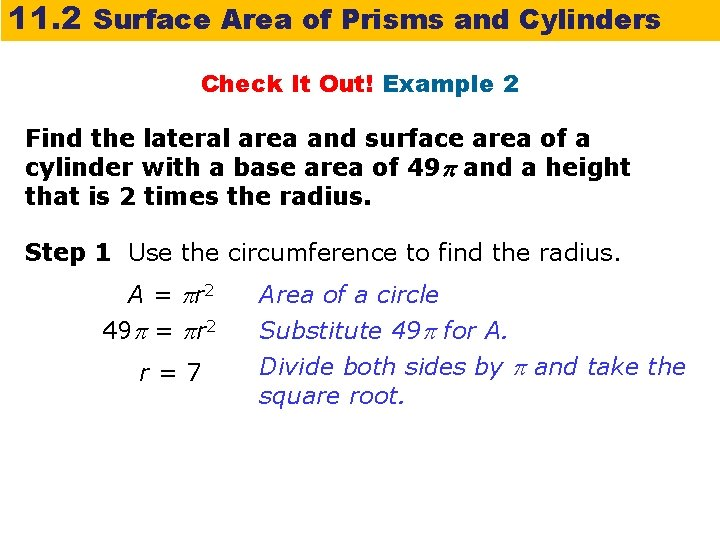11. 2 Surface Area of Prisms and Cylinders Check It Out! Example 2 Find the lateral area and surface area of a cylinder with a base area of 49 and a height that is 2 times the radius. Step 1 Use the circumference to find the radius. A = r 2 49 = r 2 r=7 Area of a circle Substitute 49 for A. Divide both sides by and take the square root.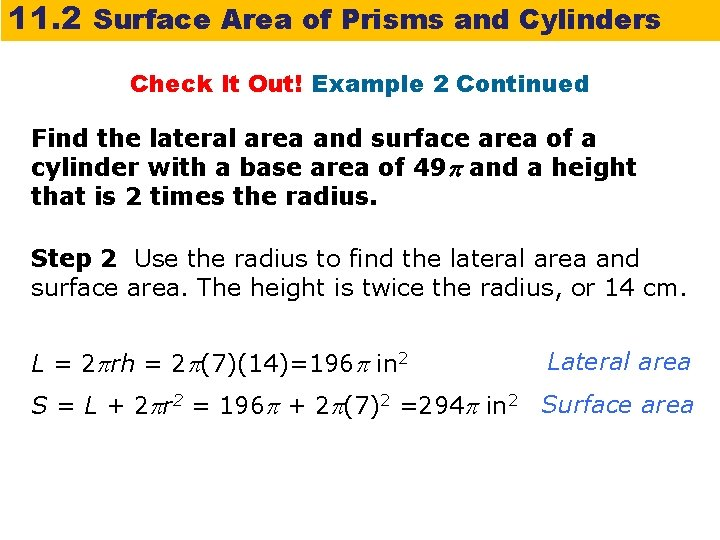11. 2 Surface Area of Prisms and Cylinders Check It Out! Example 2 Continued Find the lateral area and surface area of a cylinder with a base area of 49 and a height that is 2 times the radius. Step 2 Use the radius to find the lateral area and surface area. The height is twice the radius, or 14 cm. L = 2 rh = 2 (7)(14)=196 in 2 Lateral area S = L + 2 r 2 = 196 + 2 (7)2 =294 in 2 Surface area11. 2 Surface Area of Prisms and Cylinders Example 3: Finding Surface Areas of Composite Three-Dimensional Figures Find the surface area of the composite figure.11. 2 Surface Area of Prisms and Cylinders Example 3 Continued The surface area of the rectangular prism is. A right triangular prism is added to the rectangular prism. The surface area of the triangular prism is. Two copies of the rectangular prism base are removed. The area of the base is B = 2(4) = 8 cm 2.11. 2 Surface Area of Prisms and Cylinders Example 3 Continued The surface area of the composite figure is the sum of the areas of all surfaces on the exterior of the figure. S = (rectangular prism surface area) + (triangular prism surface area) – 2(rectangular prism base area) S = 52 + 36 – 2(8) = 72 cm 2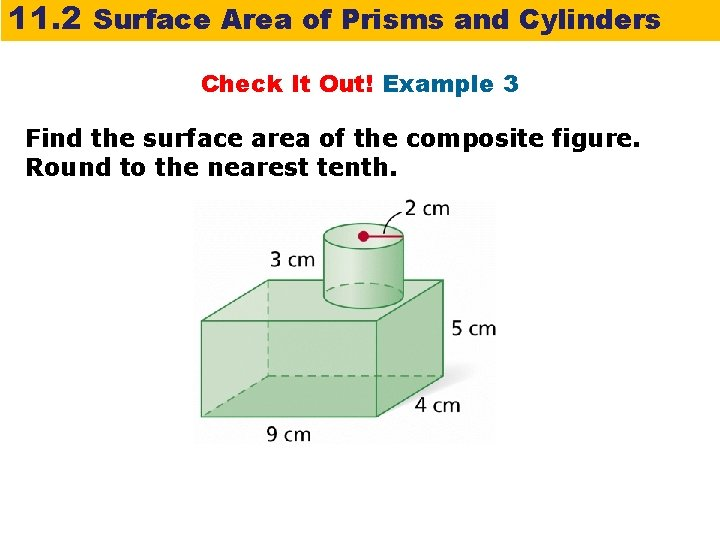11. 2 Surface Area of Prisms and Cylinders Check It Out! Example 3 Find the surface area of the composite figure. Round to the nearest tenth.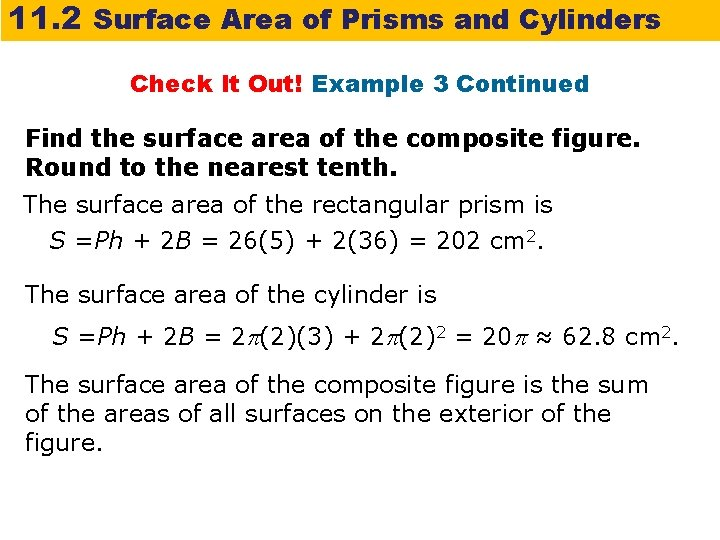11. 2 Surface Area of Prisms and Cylinders Check It Out! Example 3 Continued Find the surface area of the composite figure. Round to the nearest tenth. The surface area of the rectangular prism is S =Ph + 2 B = 26(5) + 2(36) = 202 cm 2. The surface area of the cylinder is S =Ph + 2 B = 2 (2)(3) + 2 (2)2 = 20 ≈ 62. 8 cm 2. The surface area of the composite figure is the sum of the areas of all surfaces on the exterior of the figure.11. 2 Surface Area of Prisms and Cylinders Check It Out! Example 3 Continued Find the surface area of the composite figure. Round to the nearest tenth. S = (rectangular surface area) + (cylinder surface area) – 2(cylinder base area) S = 202 + 62. 8 — 2( )(22) = 239. 7 cm 2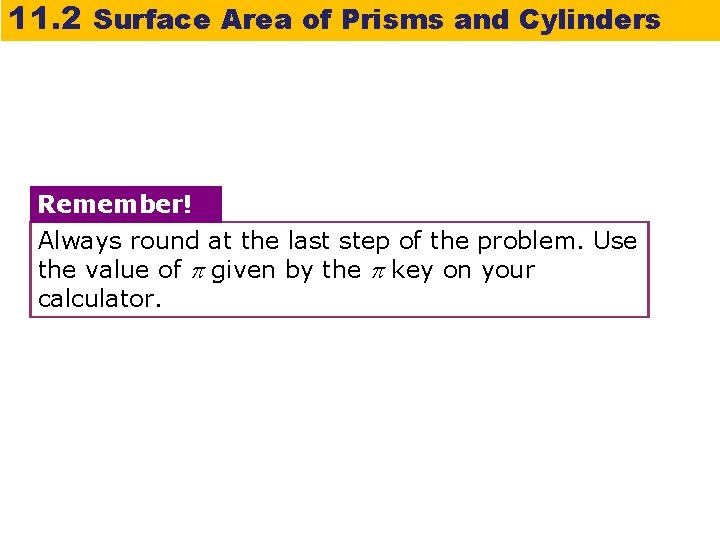11. 2 Surface Area of Prisms and Cylinders Remember! Always round at the last step of the problem. Use the value of given by the key on your calculator.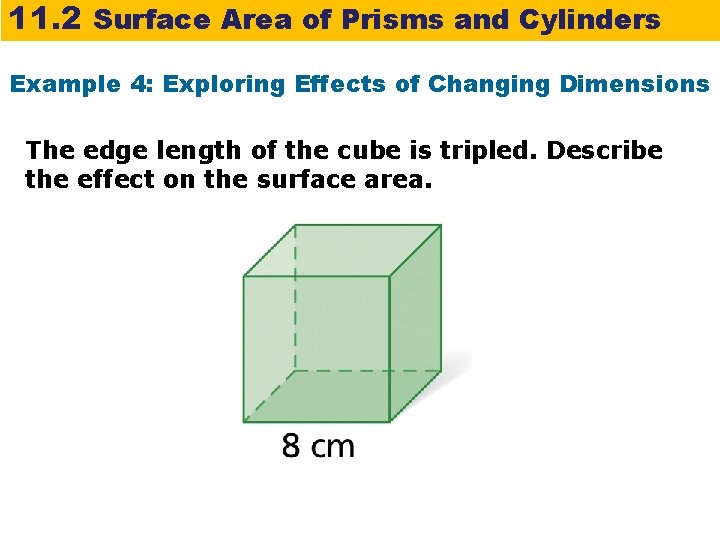11. 2 Surface Area of Prisms and Cylinders Example 4: Exploring Effects of Changing Dimensions The edge length of the cube is tripled. Describe the effect on the surface area.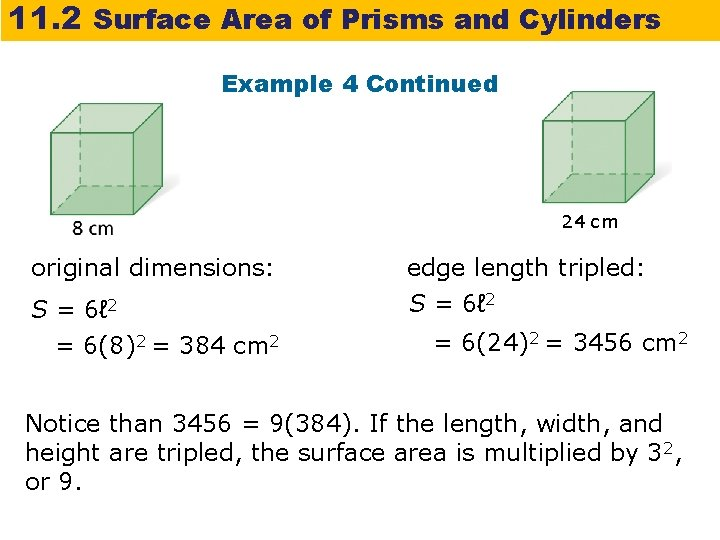11. 2 Surface Area of Prisms and Cylinders Example 4 Continued 24 cm original dimensions: edge length tripled: S = 6ℓ 2 = 6(8)2 = 384 cm 2 S = 6ℓ 2 = 6(24)2 = 3456 cm 2 Notice than 3456 = 9(384). If the length, width, and height are tripled, the surface area is multiplied by 32, or 9.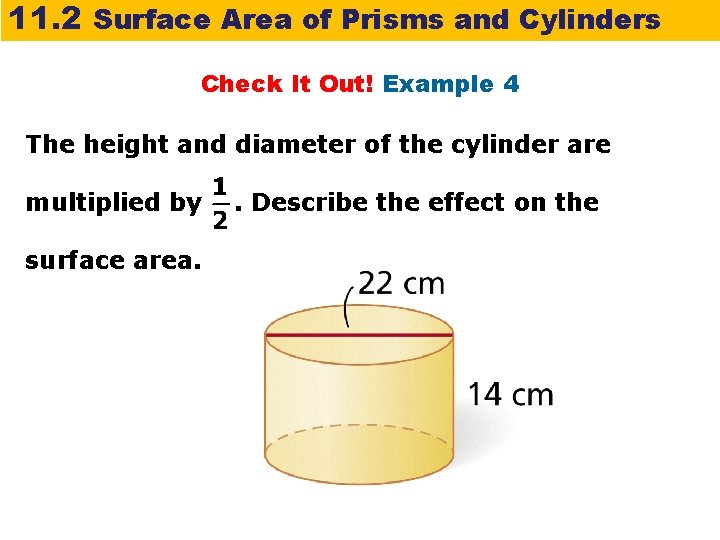11. 2 Surface Area of Prisms and Cylinders Check It Out! Example 4 The height and diameter of the cylinder are multiplied by surface area. . Describe the effect on the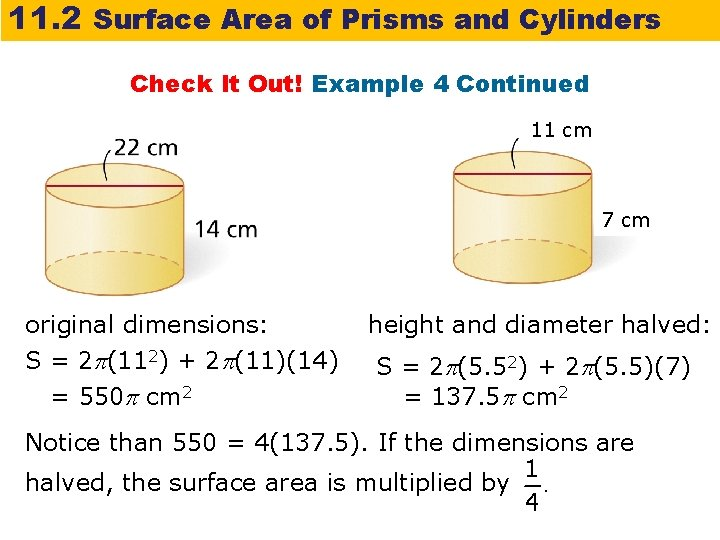11. 2 Surface Area of Prisms and Cylinders Check It Out! Example 4 Continued 11 cm 7 cm original dimensions: S = 2 (112) + 2 (11)(14) = 550 cm 2 height and diameter halved: S = 2 (5. 52) + 2 (5. 5)(7) = 137. 5 cm 2 Notice than 550 = 4(137. 5). If the dimensions are halved, the surface area is multiplied by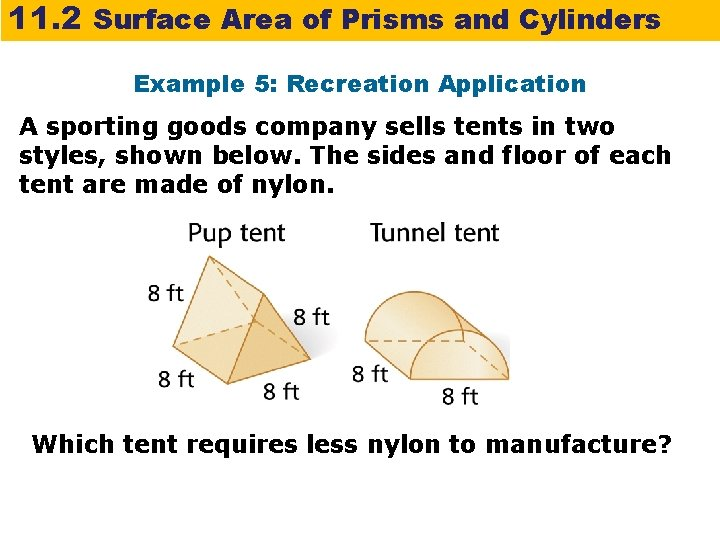11. 2 Surface Area of Prisms and Cylinders Example 5: Recreation Application A sporting goods company sells tents in two styles, shown below. The sides and floor of each tent are made of nylon. Which tent requires less nylon to manufacture?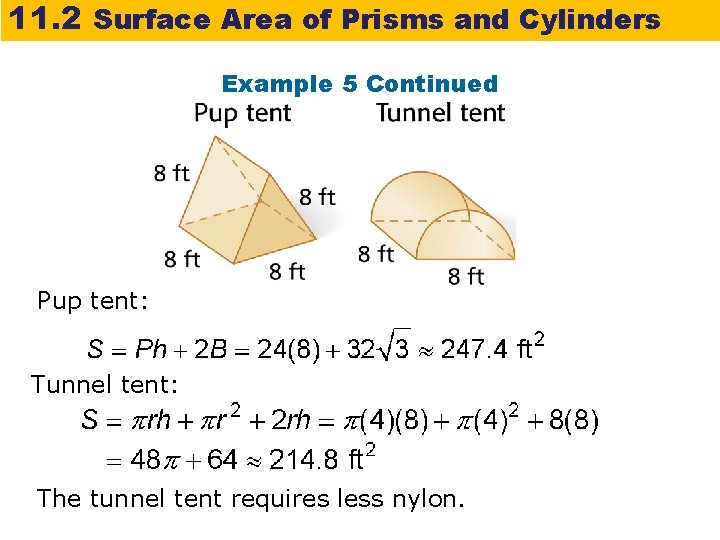11. 2 Surface Area of Prisms and Cylinders Example 5 Continued Pup tent: Tunnel tent: The tunnel tent requires less nylon.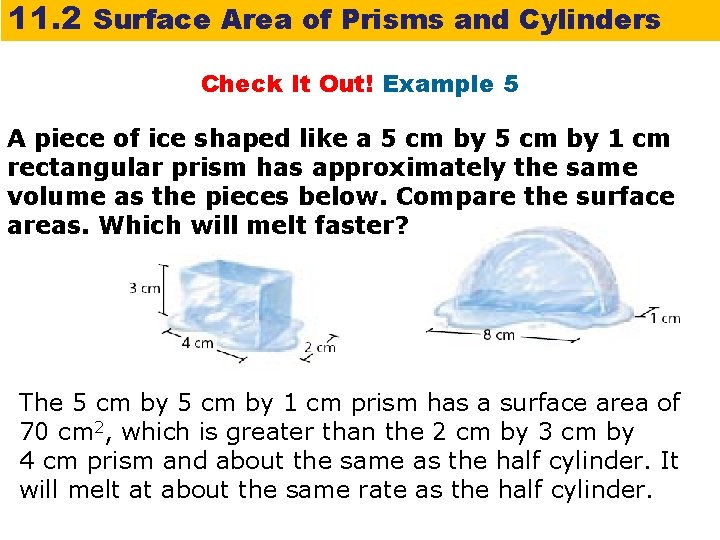11. 2 Surface Area of Prisms and Cylinders Check It Out! Example 5 A piece of ice shaped like a 5 cm by 1 cm rectangular prism has approximately the same volume as the pieces below. Compare the surface areas. Which will melt faster? The 5 cm by 1 cm prism has a surface area of 70 cm 2, which is greater than the 2 cm by 3 cm by 4 cm prism and about the same as the half cylinder. It will melt at about the same rate as the half cylinder.